# NCERT Exemplar Class 12 Physics Solutions for Chapter 14 Semiconductor Electronics Materials Devices and Simple Circuits

NCERT Class 12 Physics Exemplar Solutions for Chapter 14 Semiconductor Electronics: Materials, Devices and Simple Circuits, provided here, are prepared by experts after extensive research on every topic to provide apt and authentic information to the students. If you go through previous years’ question papers, you will get a clear picture of questions in the exemplar book that are asked in the board examination. To score good marks, students need to solve and get well-versed with the exemplar questions and problems given in this exemplar book.

The NCERT Class 12 Physics Exemplar for Chapter 14 PDF presents different varieties of questions, like MCQs, fill in the blanks, match the following, true or false, short answer questions, along with numerical problems, important formulas, exercises and assignments. It also helps students to understand the concepts clearly and memorise topics for a long period of time.

## Download the PDF of the NCERT Exemplar for Class 12 Physics Chapter 14 Semiconductor Electronics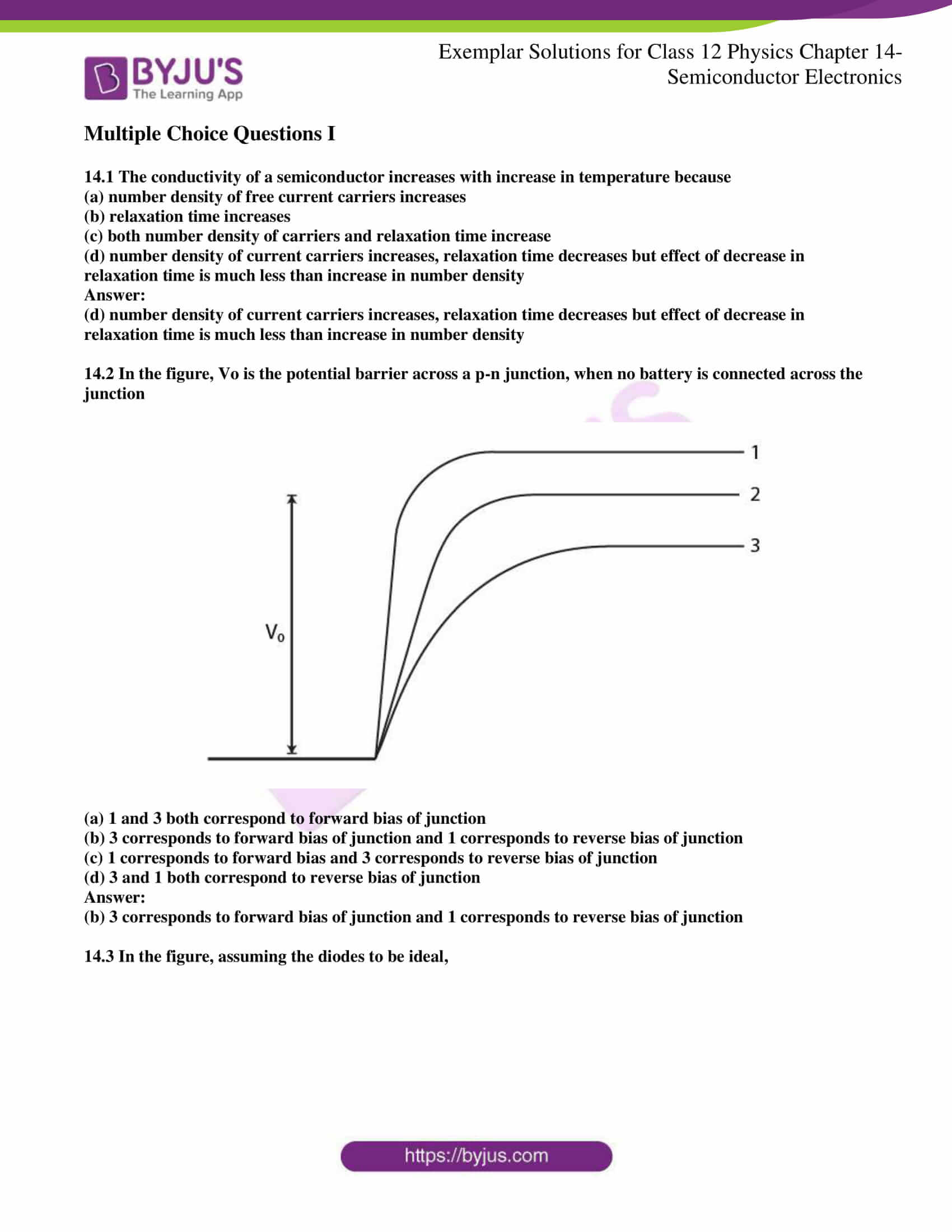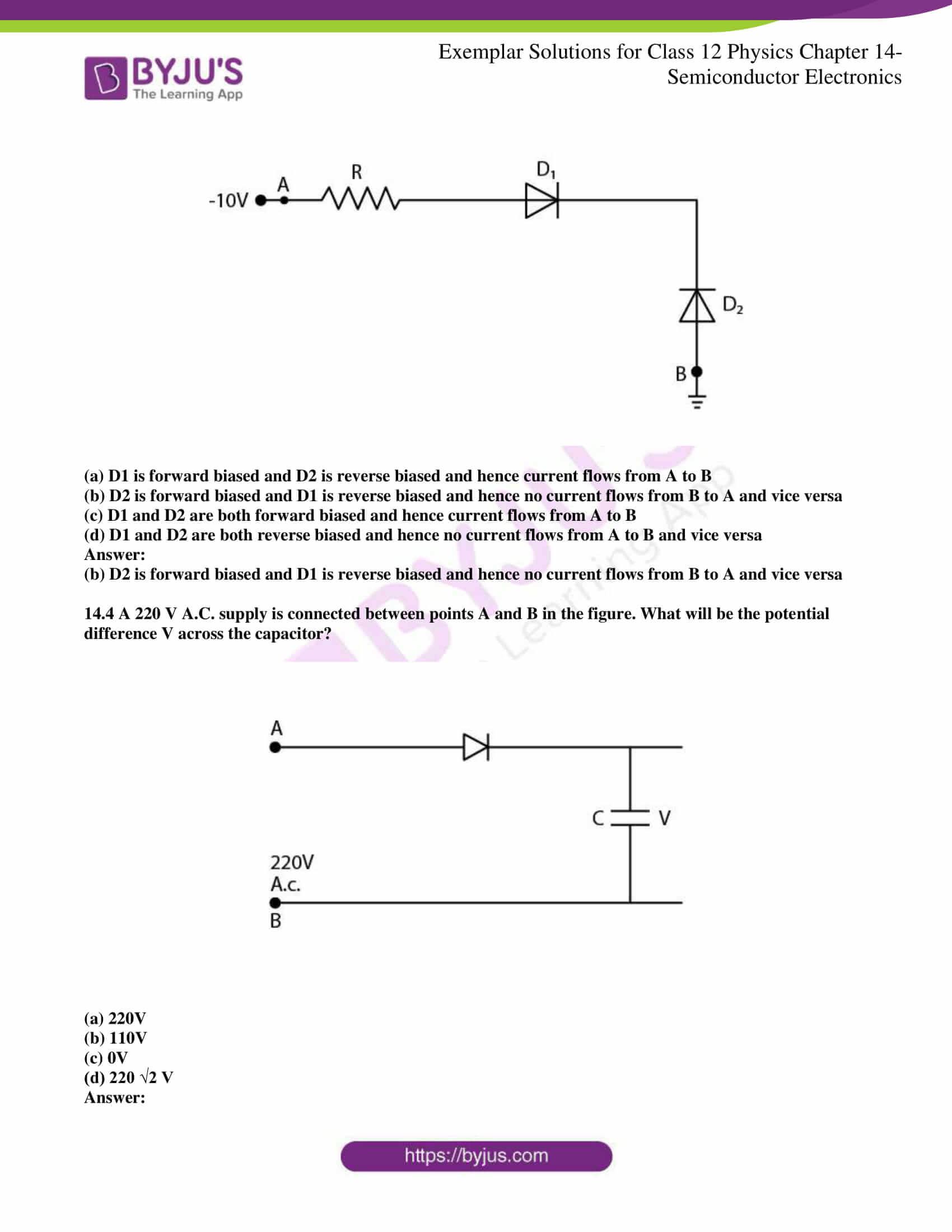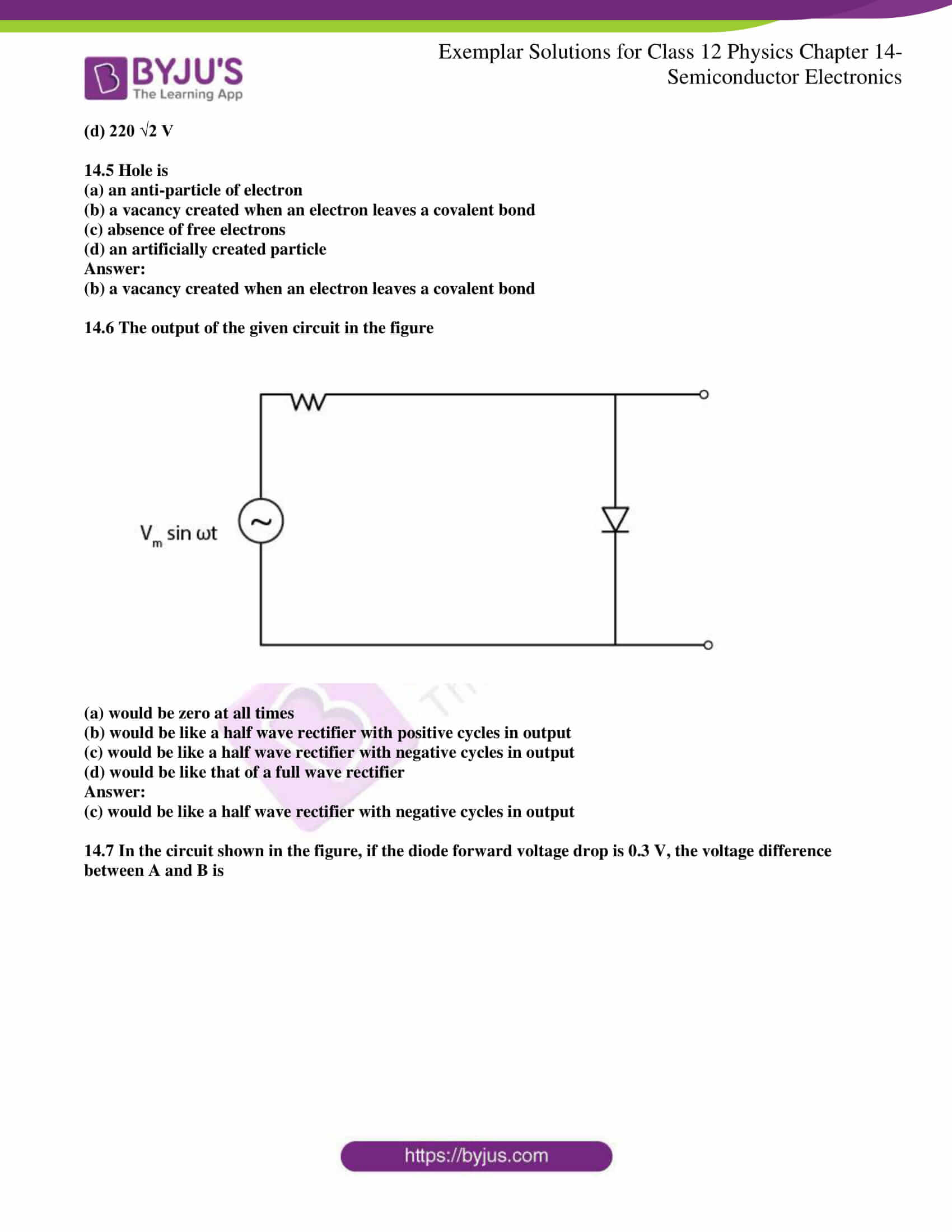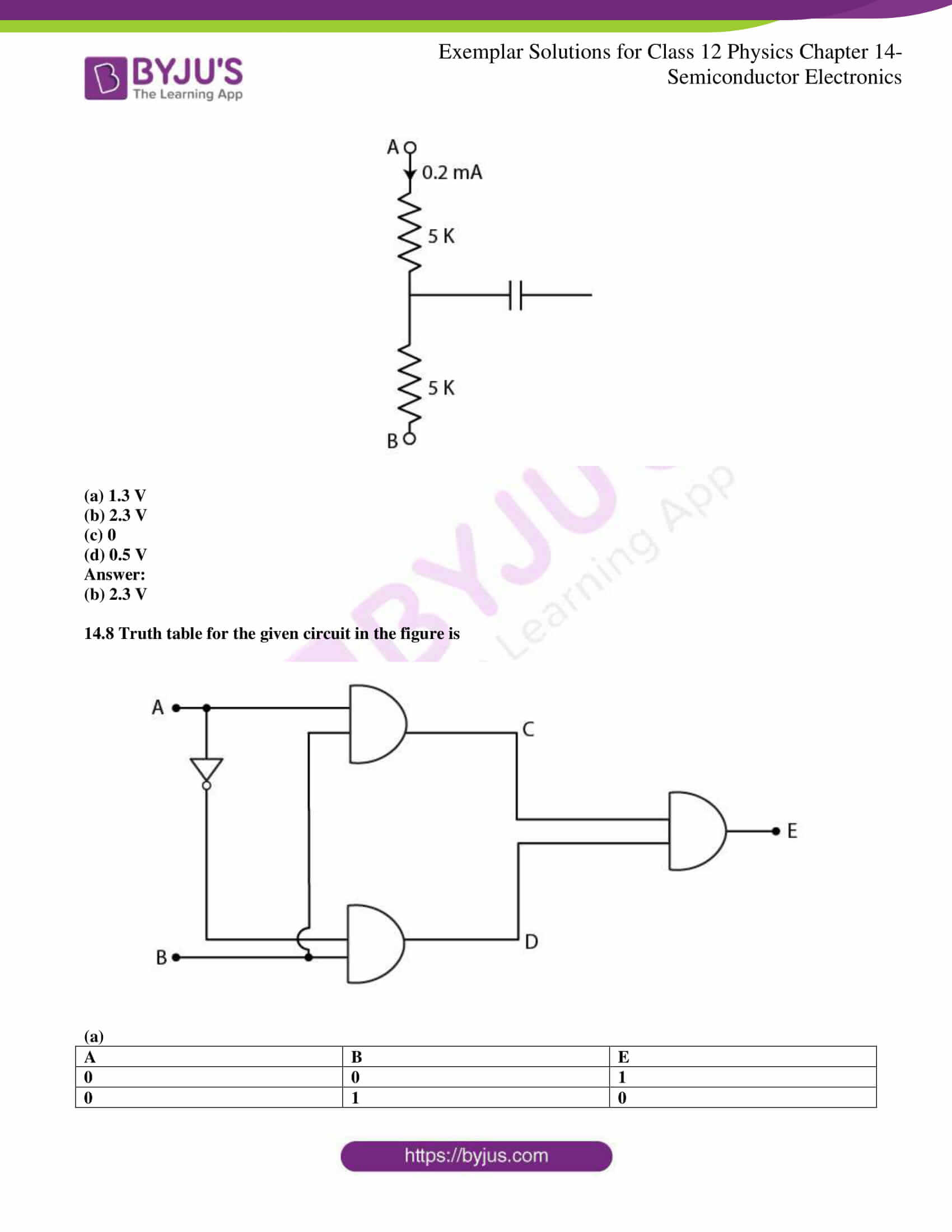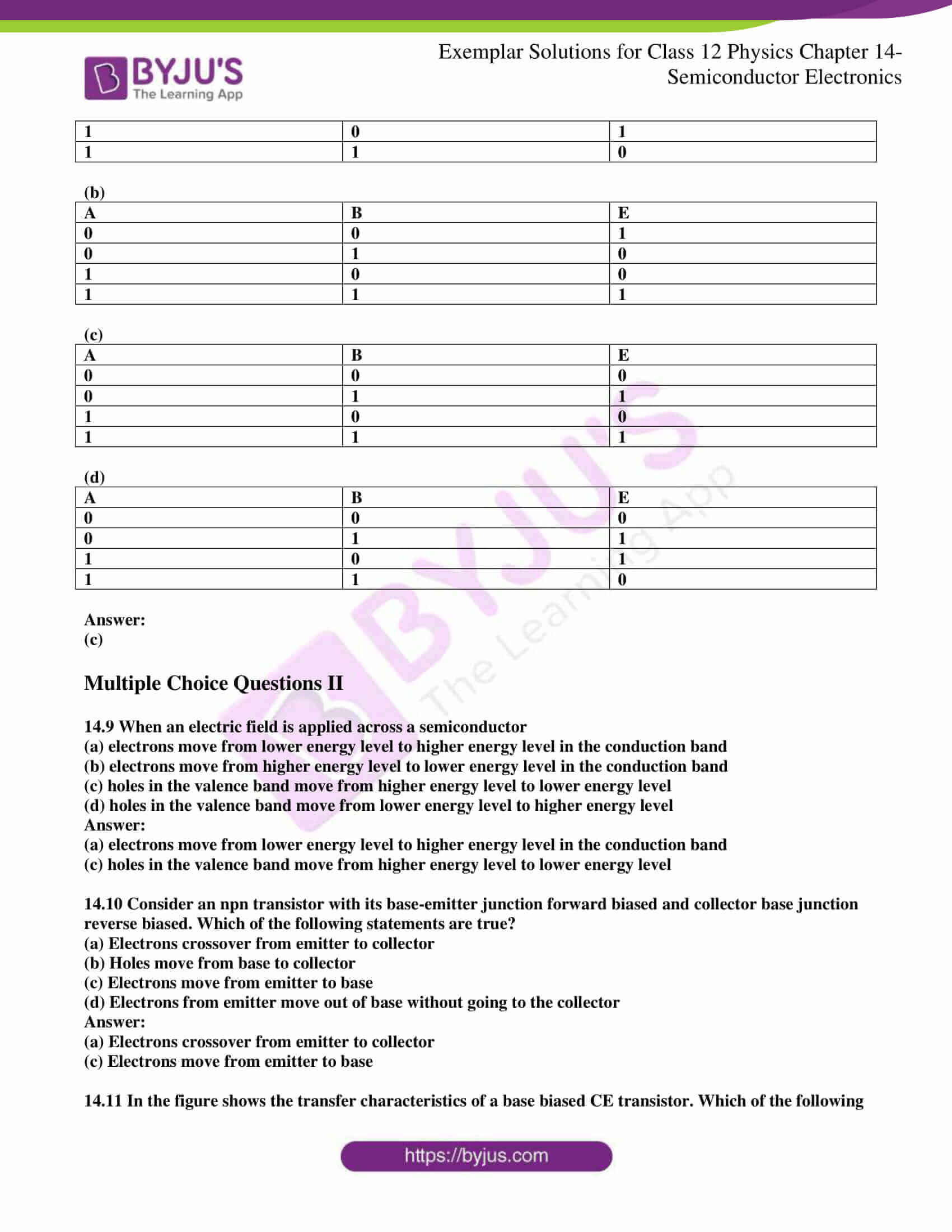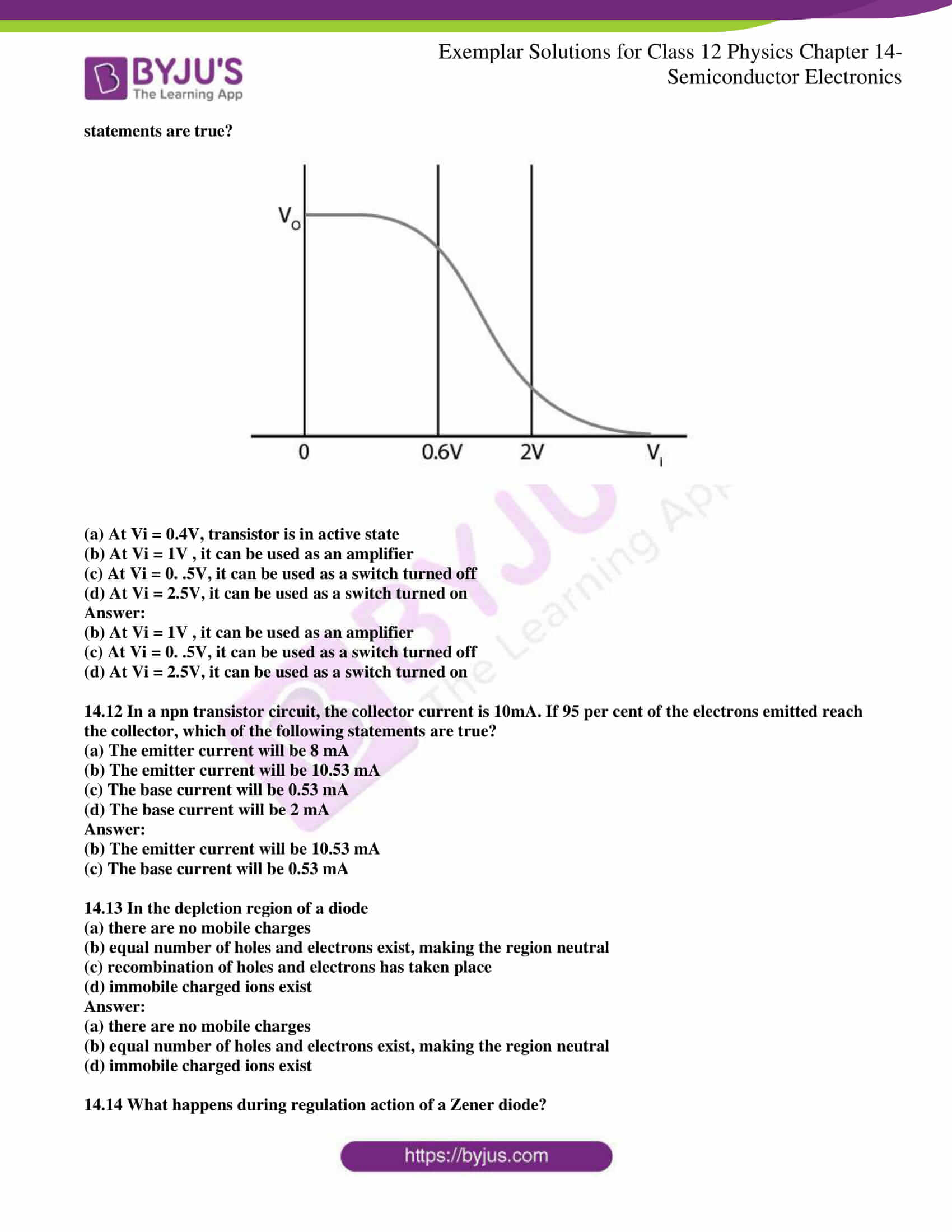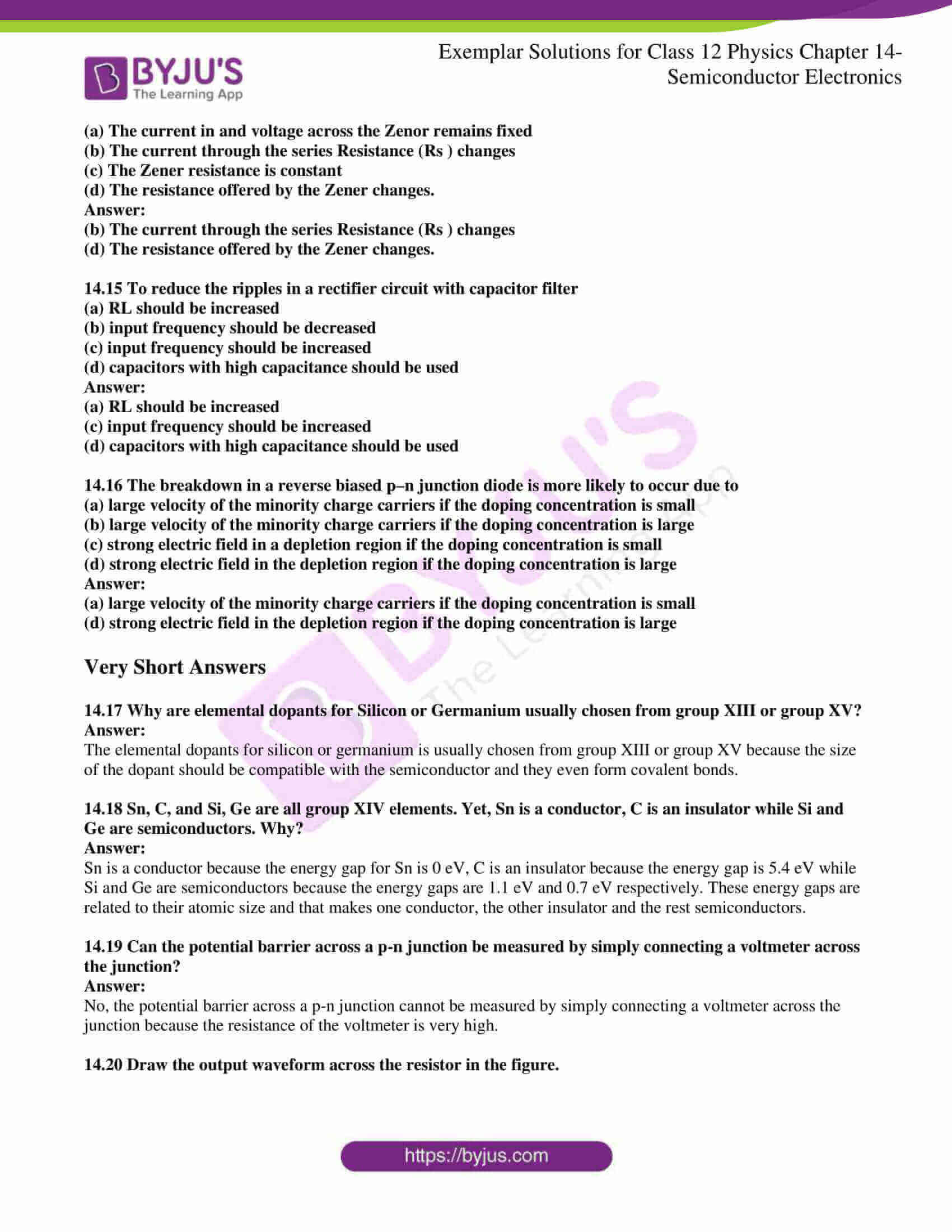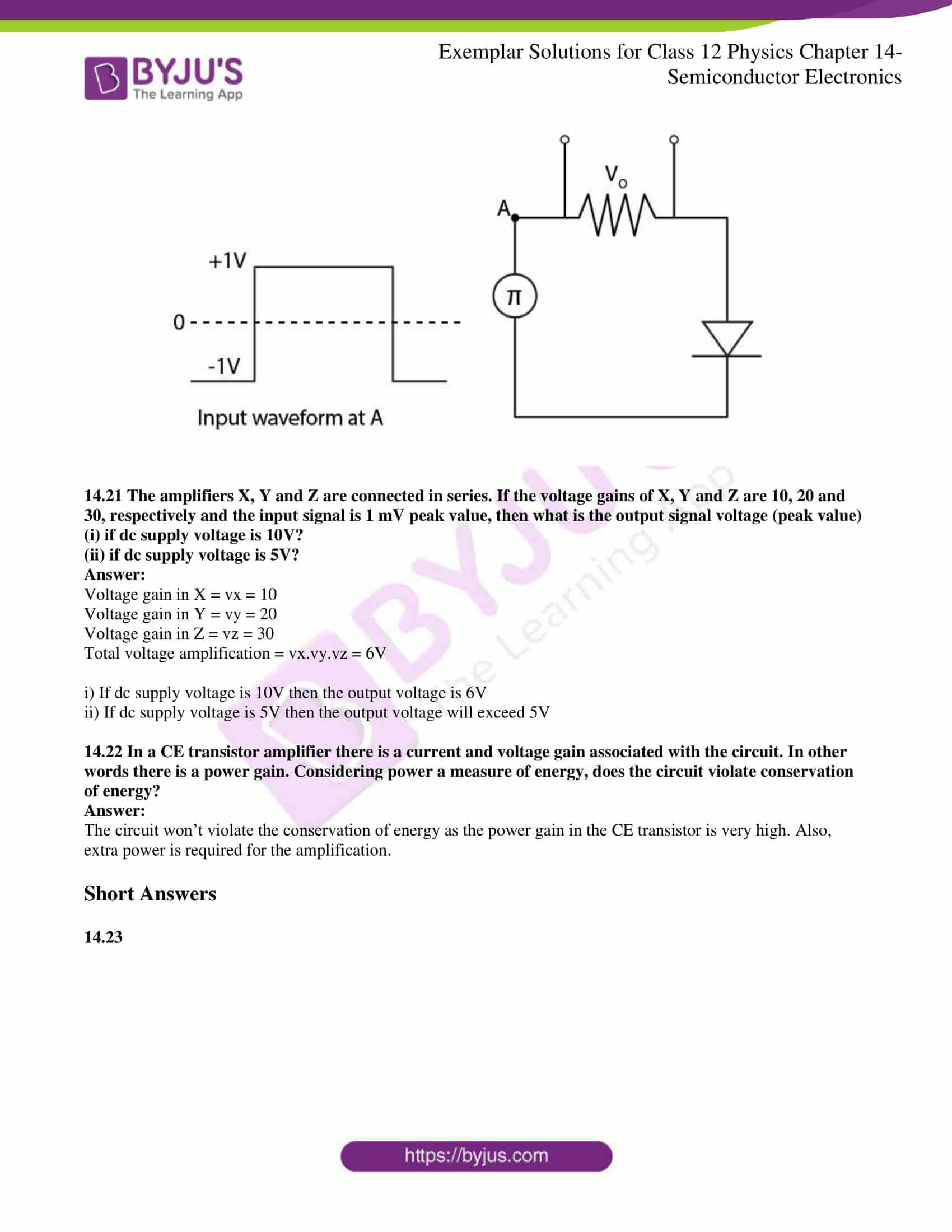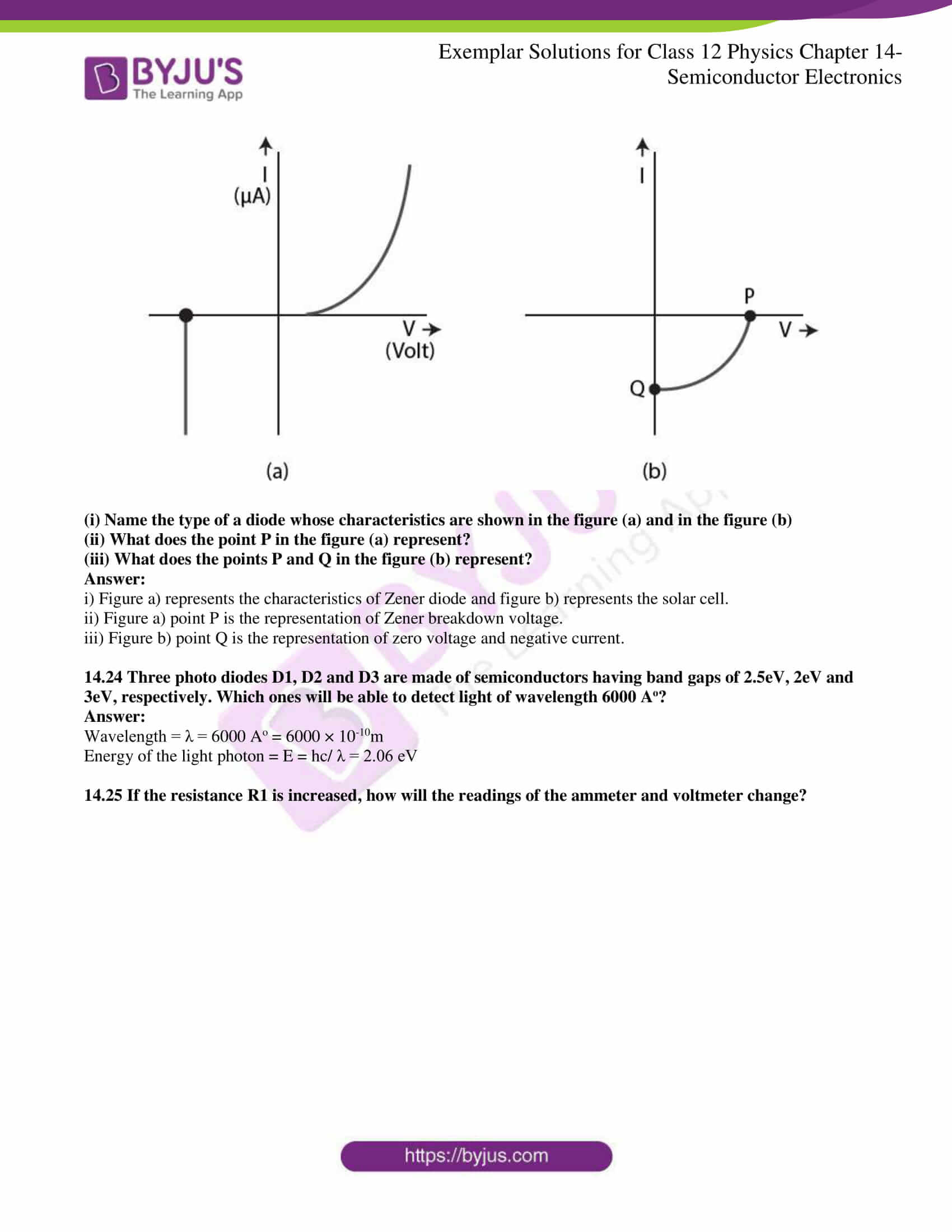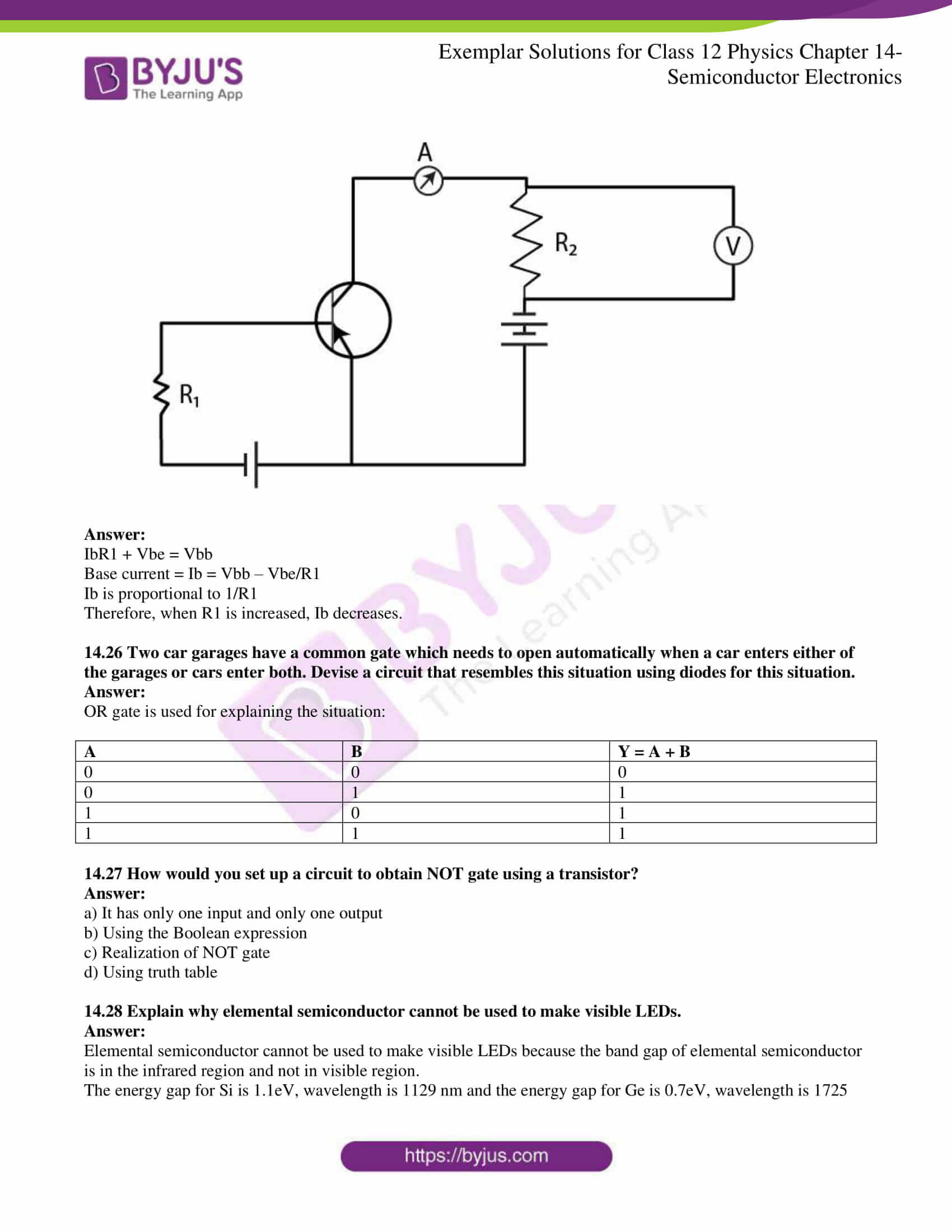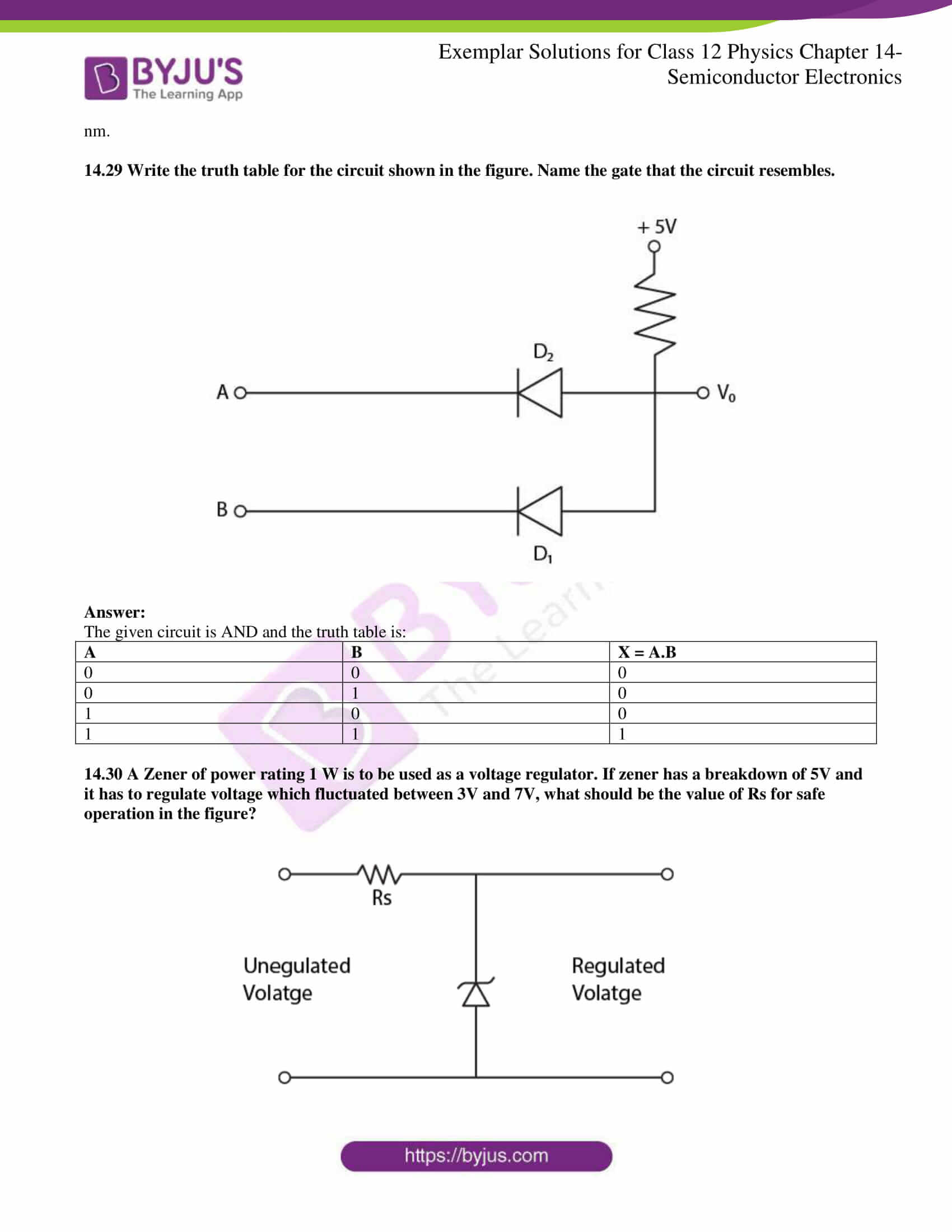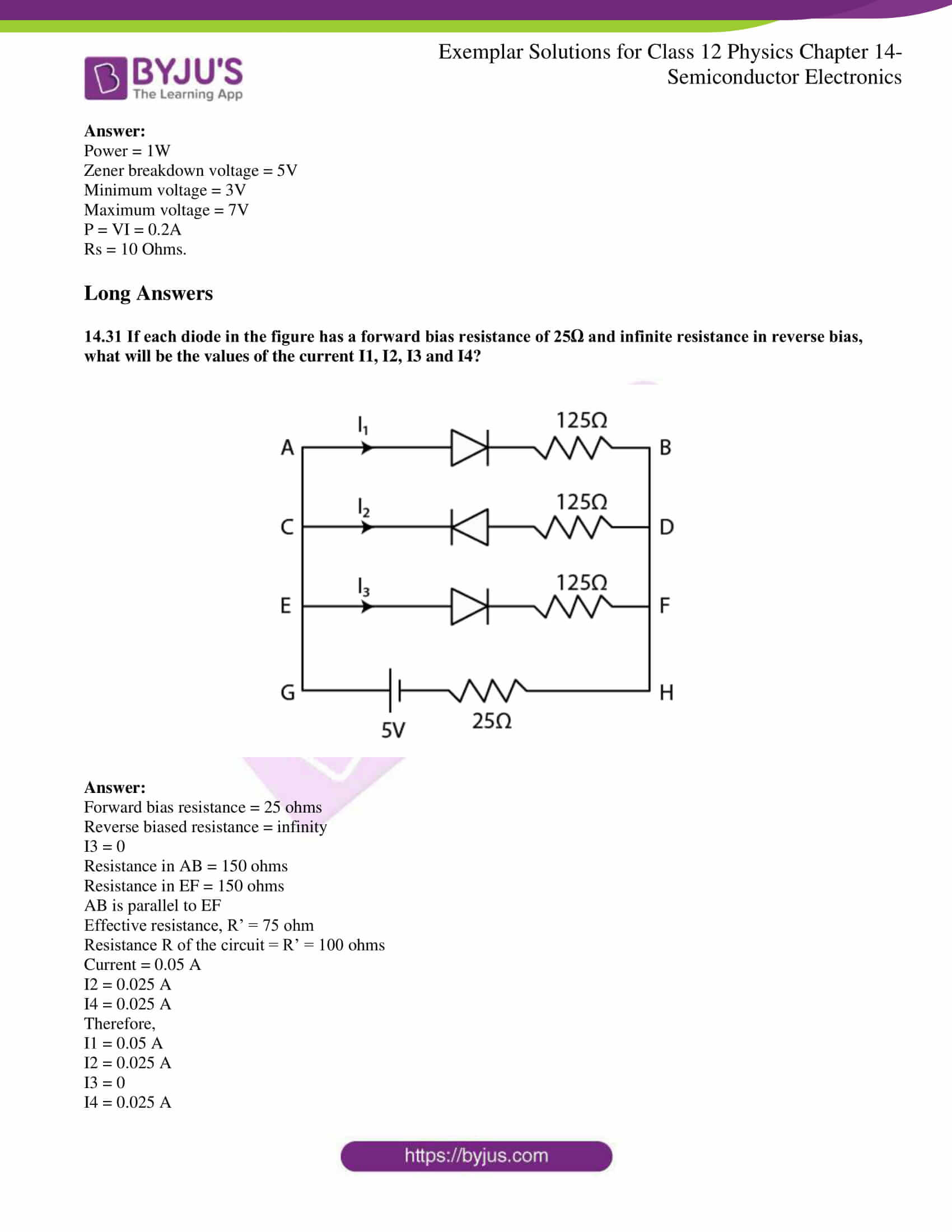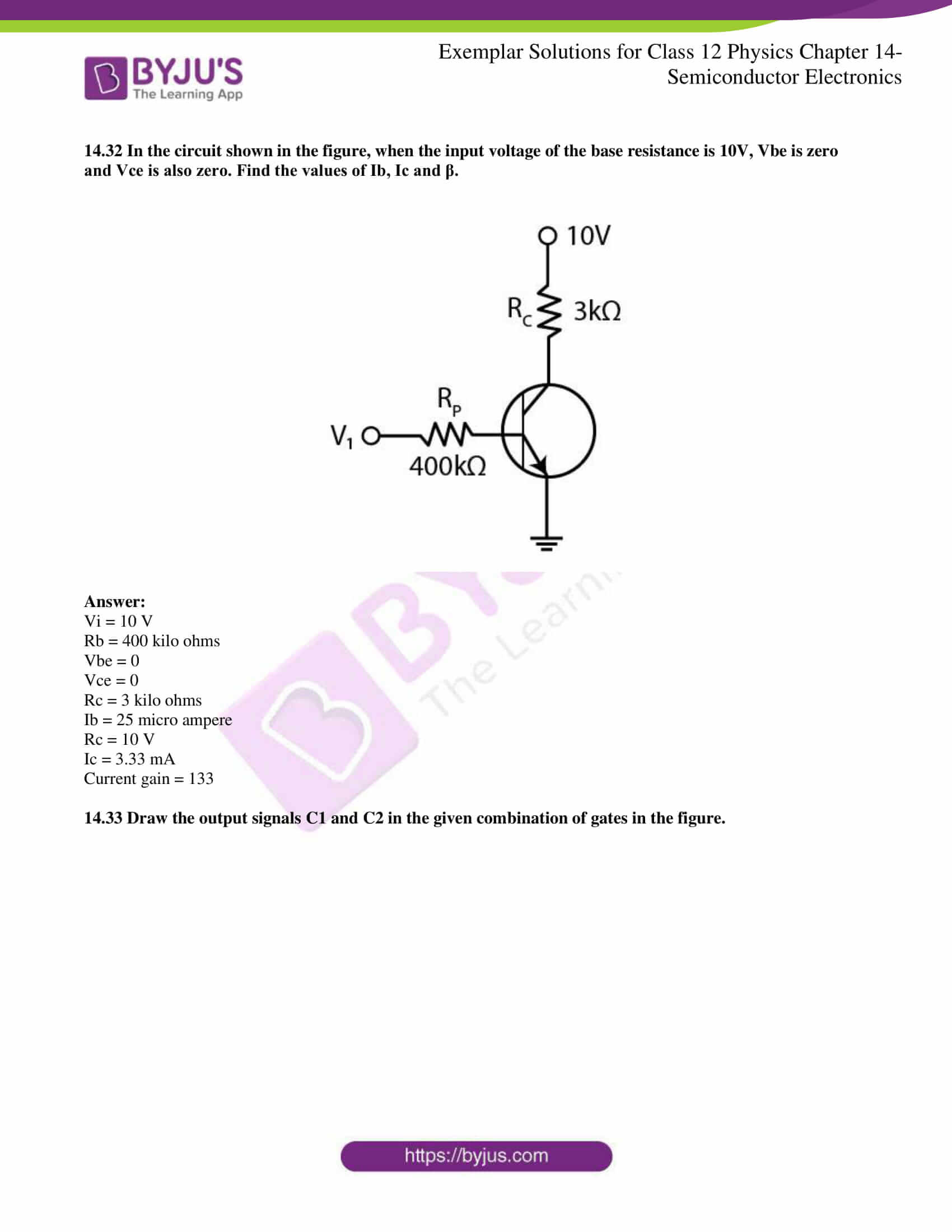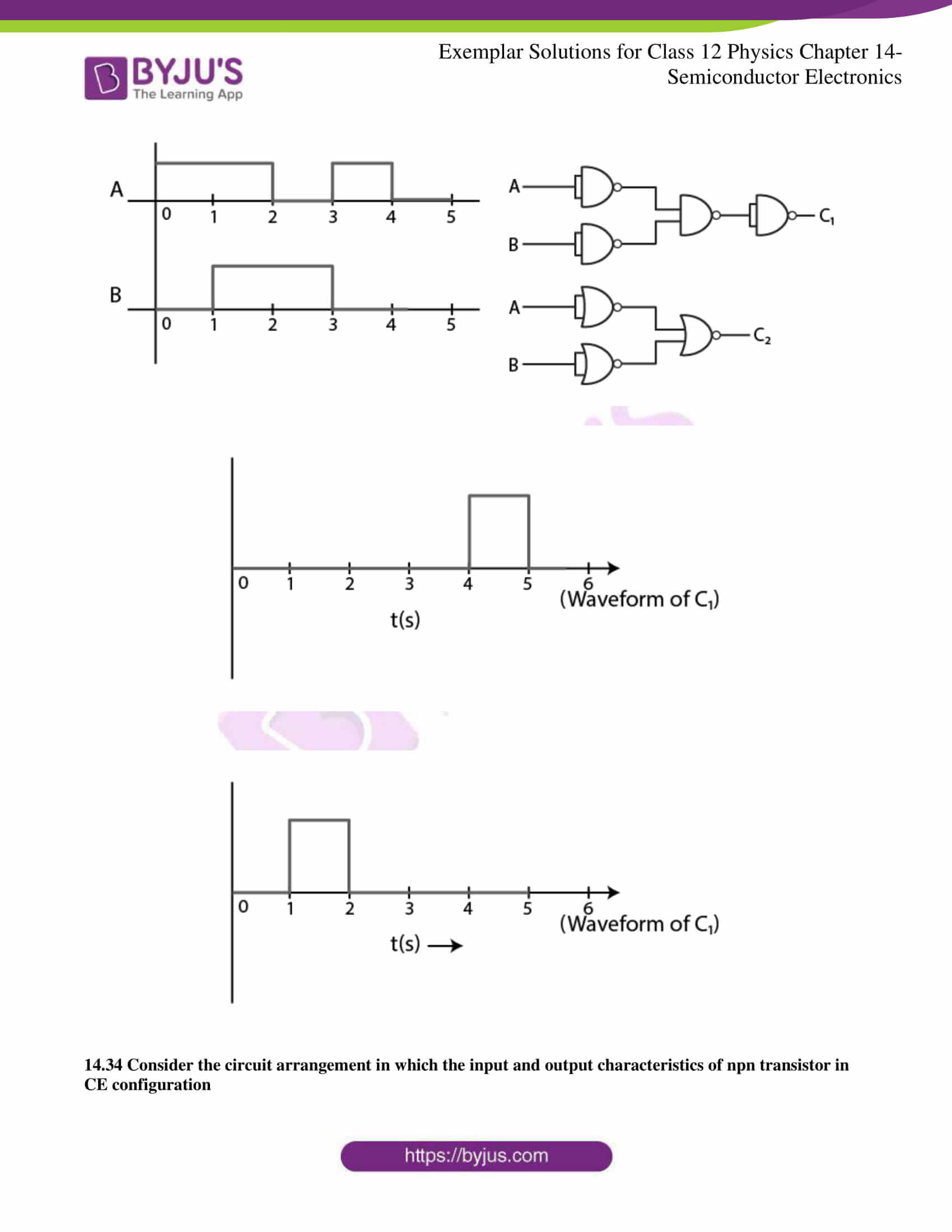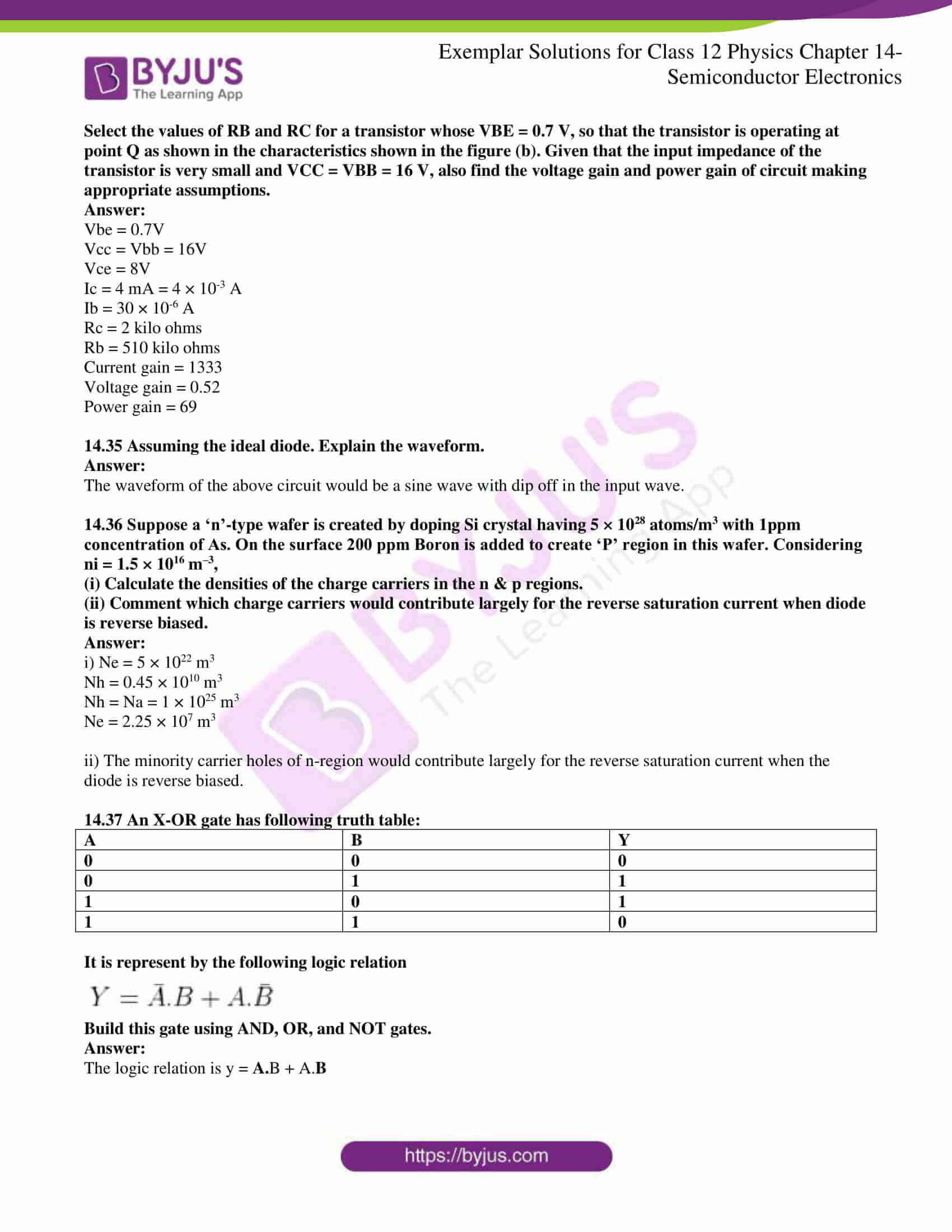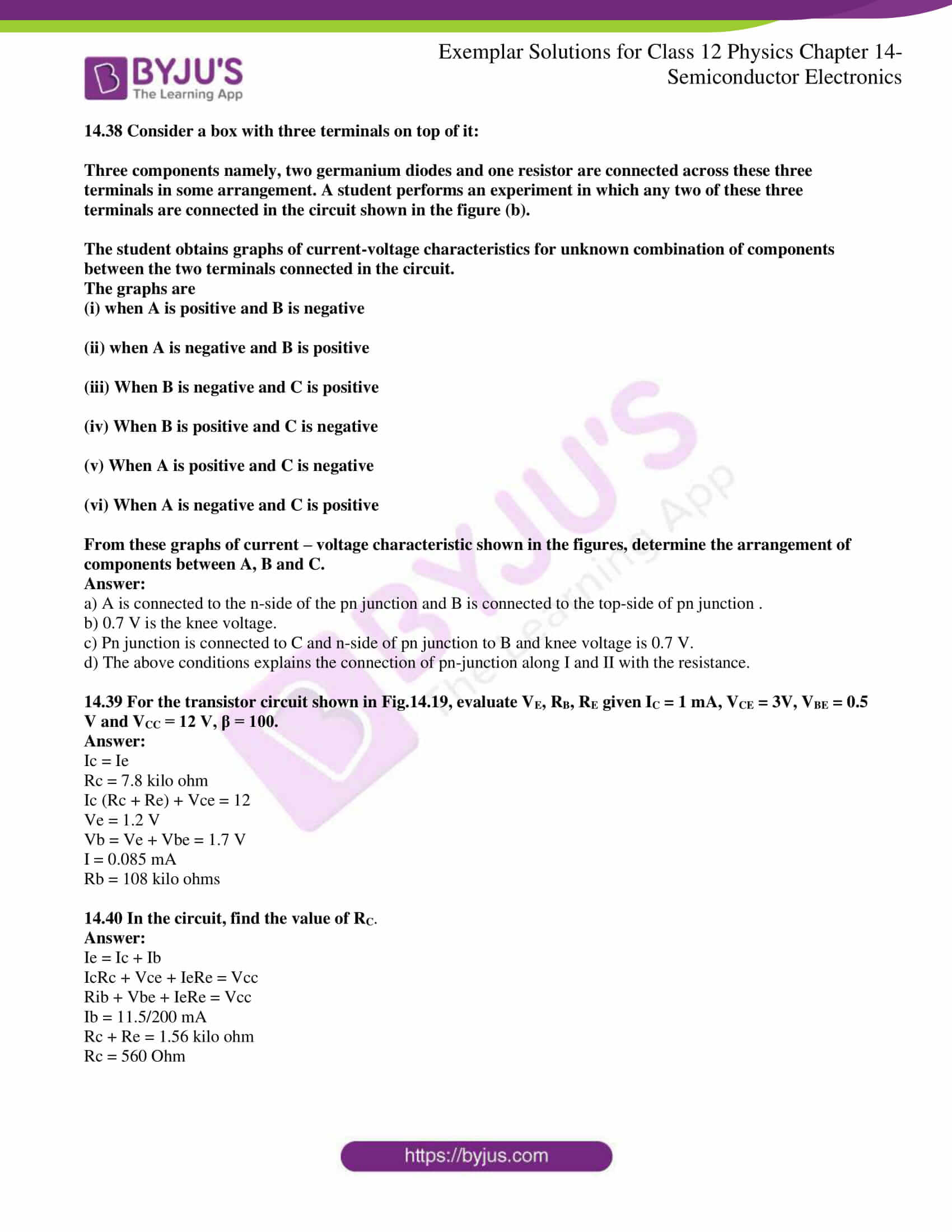### Multiple Choice Questions I

14.1 The conductivity of a semiconductor increases with increase in temperature because

(a) number density of free current carriers increases

(b) relaxation time increases

(c) both number density of carriers and relaxation time increase

(d) number density of current carriers increases, relaxation time decreases but the effect of a decrease in relaxation time is much less than the increase in number density

(d) number density of current carriers increases, relaxation time decreases but the effect of a decrease in relaxation time is much less than the increase in number density

14.2 In the figure, Vo is the potential barrier across a p-n junction, when no battery is connected across the junction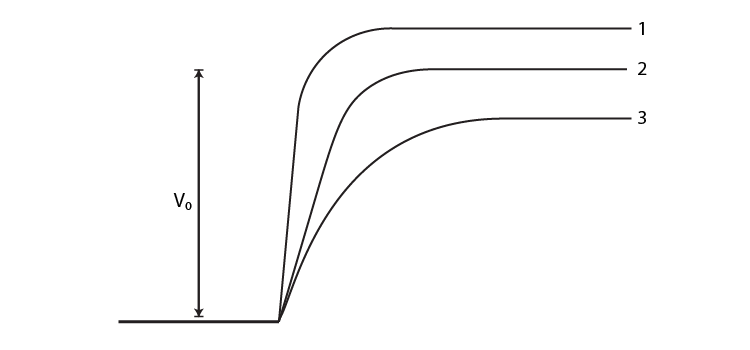(a) 1 and 3 both correspond to forward bias of junction

(b) 3 corresponds to forward bias of junction and 1 corresponds to reverse bias of junction

(c) 1 corresponds to forward bias and 3 corresponds to reverse bias of junction

(d) 3 and 1 both correspond to reverse bias of junction

(b) 3 corresponds to forward bias of junction and 1 corresponds to reverse bias of junction

14.3 In the figure, assuming the diodes to be ideal,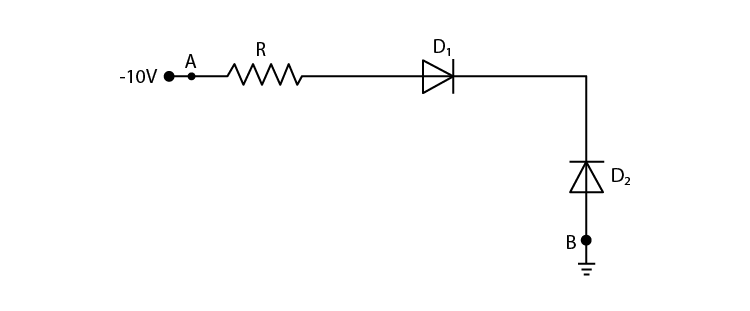(a) D1 is forward biased and D2 is reverse biased and hence current flows from A to B

(b) D2 is forward biased and D1 is reverse biased and hence no current flows from B to A and vice versa

(c) D1 and D2 are both forward biased and hence current flows from A to B

(d) D1 and D2 are both reverse biased and hence no current flows from A to B and vice versa

(b) D2 is forward biased and D1 is reverse biased and hence no current flows from B to A and vice versa

14.4 A 220 V A.C. supply is connected between points A and B in the figure. What will be the potential difference V across the capacitor?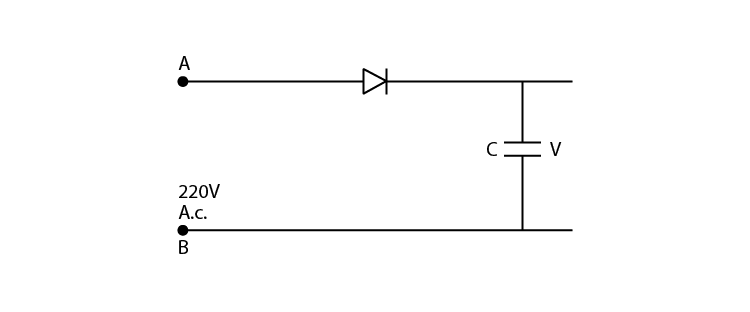(a) 220V

(b) 110V

(c) 0V

(d) 220 √2 V

(d) 220 √2 V

14.5 Hole is

(a) an anti-particle of the electron

(b) a vacancy created when an electron leaves a covalent bond

(c) absence of free electrons

(d) an artificially created particle

(b) a vacancy created when an electron leaves a covalent bond

14.6 The output of the given circuit in the figure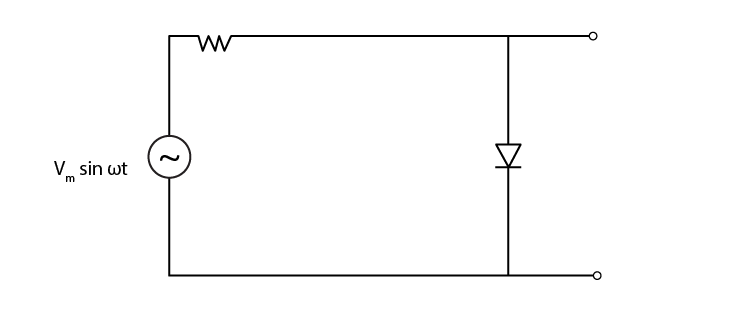(a) would be zero at all times

(b) would be like a half-wave rectifier with positive cycles in output

(c) would be like a half-wave rectifier with negative cycles in output

(d) would be like that of a full-wave rectifier

(c) would be like a half-wave rectifier with negative cycles in output

14.7 In the circuit shown in the figure, if the diode forward voltage drop is 0.3 V, the voltage difference between A and B is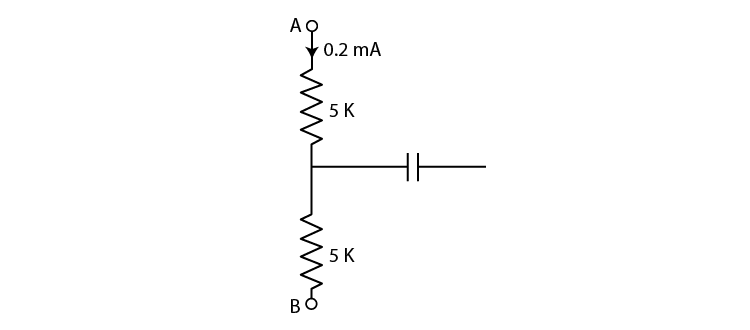(a) 1.3 V

(b) 2.3 V

(c) 0

(d) 0.5 V

(b) 2.3 V

14.8 Truth table for the given circuit in the figure is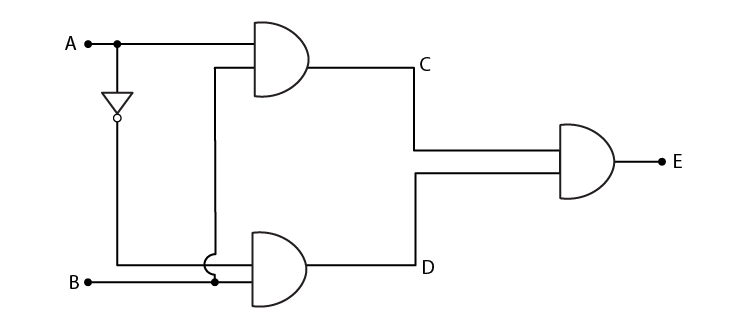(a)

 A B E 0 0 1 0 1 0 1 0 1 1 1 0

(b)

 A B E 0 0 1 0 1 0 1 0 0 1 1 1

(c)

 A B E 0 0 0 0 1 1 1 0 0 1 1 1

(d)

 A B E 0 0 0 0 1 1 1 0 1 1 1 0

(c)

 A B E 0 0 0 0 1 1 1 0 0 1 1 1

### Multiple Choice Questions II

14.9 When an electric field is applied across a semiconductor

(a) electrons move from lower energy level to higher energy level in the conduction band

(b) electrons move from higher energy level to lower energy level in the conduction band

(c) holes in the valence band move from higher energy level to lower energy level

(d) holes in the valence band move from lower energy level to higher energy level

(a) electrons move from lower energy level to higher energy level in the conduction band

(c) holes in the valence band move from higher energy level to lower energy level

14.10 Consider an NPN transistor with its base-emitter junction forward biased and collector-base junction reverse biased. Which of the following statements are true?

(a) Electrons crossover from emitter to collector

(b) Holes move from base to collector

(c) Electrons move from emitter to base

(d) Electrons from emitter move out of base without going to the collector

(a) Electrons crossover from emitter to collector

(c) Electrons move from emitter to base

14.11 In the figure shows the transfer characteristics of a base biased CE transistor. Which of the following statements are true?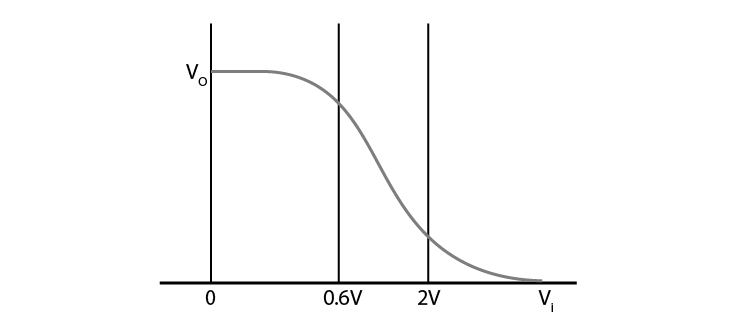(a) At Vi = 0.4V, transistor is in active state

(b) At Vi = 1V, it can be used as an amplifier

(c) At Vi = 0. .5V, it can be used as a switch turned off

(d) At Vi = 2.5V, it can be used as a switch turned on

(b) At Vi = 1V, it can be used as an amplifier

(c) At Vi = 0. .5V, it can be used as a switch turned off

(d) At Vi = 2.5V, it can be used as a switch turned on

14.12 In an NPN transistor circuit, the collector current is 10mA. If 95 per cent of the electrons emitted reaches the collector, which of the following statements are true?

(a) The emitter current will be 8 mA

(b) The emitter current will be 10.53 mA

(c) The base current will be 0.53 mA

(d) The base current will be 2 mA

(b) The emitter current will be 10.53 mA

(c) The base current will be 0.53 mA

14.13 In the depletion region of a diode

(a) there are no mobile charges

(b) equal number of holes and electrons exist, making the region neutral

(c) recombination of holes and electrons has taken place

(d) immobile charged ions exist

(a) there are no mobile charges

(b) equal number of holes and electrons exist, making the region neutral

(d) immobile charged ions exist

14.14 What happens during regulation action of a Zener diode?

(a) The current in and voltage across the Zenor remains fixed

(b) The current through the series Resistance (Rs ) changes

(c) The Zener resistance is constant

(d) The resistance offered by the Zener changes.

(b) The current through the series Resistance (Rs ) changes

(d) The resistance offered by the Zener changes.

14.15 To reduce the ripples in a rectifier circuit with capacitor filter

(a) RL should be increased

(b) input frequency should be decreased

(c) input frequency should be increased

(d) capacitors with high capacitance should be used

(a) RL should be increased

(c) input frequency should be increased

(d) capacitors with high capacitance should be used

14.16 The breakdown in a reverse-biased p–n junction diode is more likely to occur due to

(a) large velocity of the minority charge carriers if the doping concentration is small

(b) large velocity of the minority charge carriers if the doping concentration is large

(c) strong electric field in a depletion region if the doping concentration is small

(d) strong electric field in the depletion region if the doping concentration is large

(a) large velocity of the minority charge carriers if the doping concentration is small

(d) strong electric field in the depletion region if the doping concentration is large

14.17 Why are elemental dopants for Silicon or Germanium usually chosen from group XIII or group XV?

The elemental dopants for silicon or germanium is usually chosen from group XIII or group XV because the size of the dopant should be compatible with the semiconductor and they even form covalent bonds.

14.18 Sn, C, and Si, Ge are all group XIV elements. Yet, Sn is a conductor, C is an insulator while Si and Ge are semiconductors. Why?

Sn is a conductor because the energy gap for Sn is 0 eV, C is an insulator because the energy gap is 5.4 eV while Si and Ge are semiconductors because the energy gaps are 1.1 eV and 0.7 eV respectively. These energy gaps are related to their atomic size and that makes one conductor, the other insulator and the rest semiconductors.

14.19 Can the potential barrier across a p-n junction be measured by simply connecting a voltmeter across the junction?

No, the potential barrier across a p-n junction cannot be measured by simply connecting a voltmeter across the junction because the resistance of the voltmeter is very high.

14.20 Draw the output waveform across the resistor in the figure.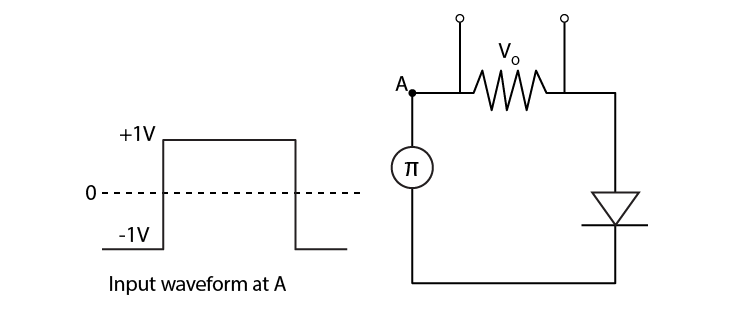14.21 The amplifiers X, Y and Z are connected in series. If the voltage gains of X, Y and Z are 10, 20 and 30, respectively and the input signal is 1 mV peak value, then what is the output signal voltage (peak value) (i) if dc supply voltage is 10V?

(ii) if dc supply voltage is 5V?

Voltage gain in X = vx = 10

Voltage gain in Y = vy = 20

Voltage gain in Z = vz = 30

Total voltage amplification = vx.vy.vz = 6V

i) If dc supply voltage is 10V then the output voltage is 6V

ii) If dc supply voltage is 5V then the output voltage will exceed 5V

14.22 In a CE transistor amplifier there is a current and voltage gain associated with the circuit. In other words, there is a power gain. Considering power a measure of energy, does the circuit violate conservation of energy?

The circuit won’t violate the conservation of energy as the power gain in the CE transistor is very high. Also, extra power is required for the amplification.

14.23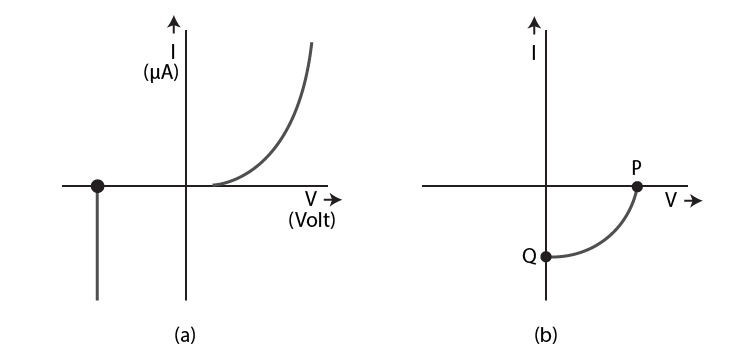(i) Name the type of a diode whose characteristics are shown in the figure (a) and in the figure (b)

(ii) What does the point P in the figure (a) represent?

(iii) What does the points P and Q in the figure (b) represent?

i) Figure a) represents the characteristics of Zener diode and figure b) represents the solar cell.

ii) Figure a) point P is the representation of Zener breakdown voltage.

iii) Figure b) point Q is the representation of zero voltage and negative current.

14.24 Three photodiodes D1, D2 and D3 are made of semiconductors having band gaps of 2.5eV, 2eV and 3eV, respectively. Which ones will be able to detect light of wavelength 6000 Ao?

Wavelength = λ = 6000 Ao = 6000 × 10-10m

Energy of the light photon = E = hc/ λ = 2.06 eV

14.25 If the resistance R1 is increased, how will the readings of the ammeter and voltmeter change?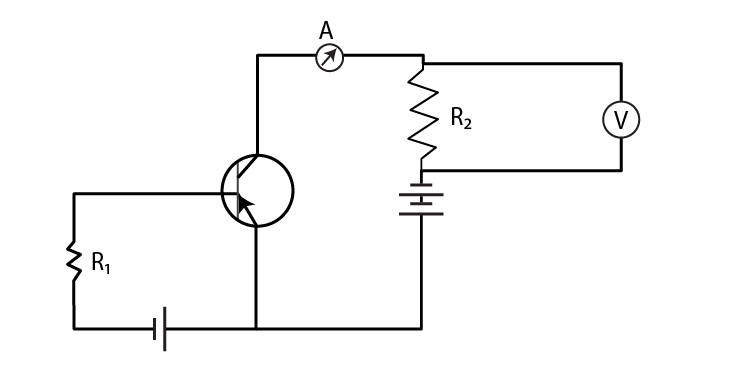IbR1 + Vbe = Vbb

Base current = Ib = Vbb – Vbe/R1

Ib is proportional to 1/R1

Therefore, when R1 is increased, Ib decreases.

14.26 Two car garages have a common gate which needs to open automatically when a car enters either of the garages or cars enter both. Devise a circuit that resembles this situation using diodes for this situation.

OR gate is used for explaining the situation:

 A B Y = A + B 0 0 0 0 1 1 1 0 1 1 1 1

14.27 How would you set up a circuit to obtain NOT gate using a transistor?

a) It has only one input and only one output

b) Using the Boolean expression

c) Realization of NOT gate

d) Using truth table

14.28 Explain why elemental semiconductor cannot be used to make visible LEDs.

Elemental semiconductor cannot be used to make visible LEDs because the bandgap of elemental semiconductor is in the infrared region and not in the visible region.

The energy gap for Si is 1.1eV, wavelength is 1129 nm and the energy gap for Ge is 0.7eV, wavelength is 1725 nm.

14.29 Write the truth table for the circuit shown in the figure. Name the gate that the circuit resembles.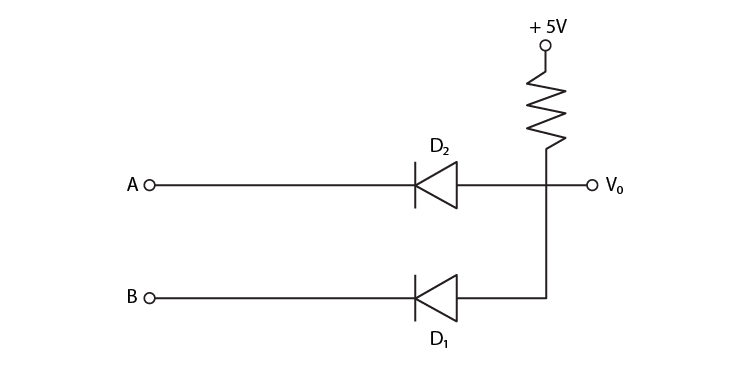The given circuit is AND and the truth table is:

 A B X = A.B 0 0 0 0 1 0 1 0 0 1 1 1

14.30 A Zener of power rating 1 W is to be used as a voltage regulator. If Zener has a breakdown of 5V and it has to regulate voltage which fluctuated between 3V and 7V, what should be the value of Rs for safe operation in the figure?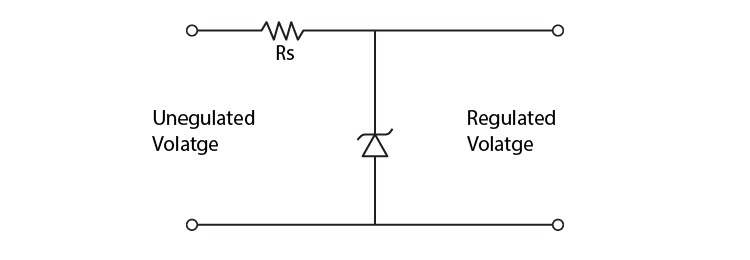Power = 1W

Zener breakdown voltage = 5V

Minimum voltage = 3V

Maximum voltage = 7V

P = VI = 0.2A

Rs = 10 Ohms.

14.31 If each diode in the figure has a forward bias resistance of 25Ω and infinite resistance in reverse bias, what will be the values of the current I1, I2, I3 and I4?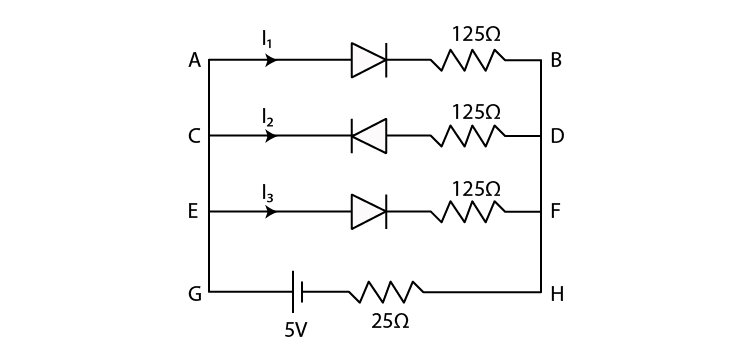Forward bias resistance = 25 ohms

Reverse biased resistance = infinity

I3 = 0

Resistance in AB = 150 ohms

Resistance in EF = 150 ohms

AB is parallel to EF

Effective resistance, R’ = 75 ohm

Resistance R of the circuit = R’ = 100 ohms

Current = 0.05 A

I2 = 0.025 A

I4 = 0.025 A

Therefore,

I1 = 0.05 A

I2 = 0.025 A

I3 = 0

I4 = 0.025 A

14.32 In the circuit shown in the figure, when the input voltage of the base resistance is 10V, Vbe is zero and Vce is also zero. Find the values of Ib, Ic and β.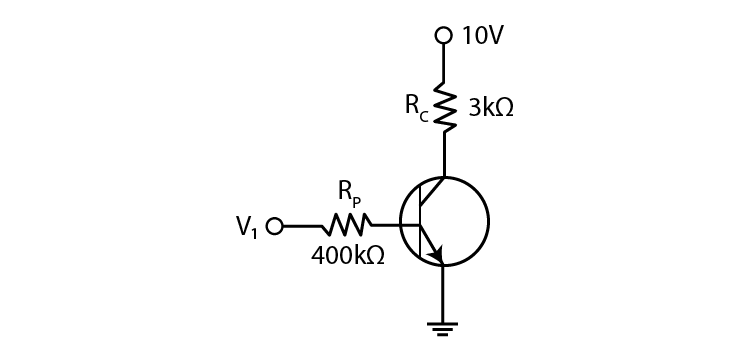Vi = 10 V

Rb = 400 kilo ohms

Vbe = 0

Vce = 0

Rc = 3 kilo ohms

Ib = 25 micro ampere

Rc = 10 V

Ic = 3.33 mA

Current gain = 133

14.33 Draw the output signals C1 and C2 in the given combination of gates in the figure.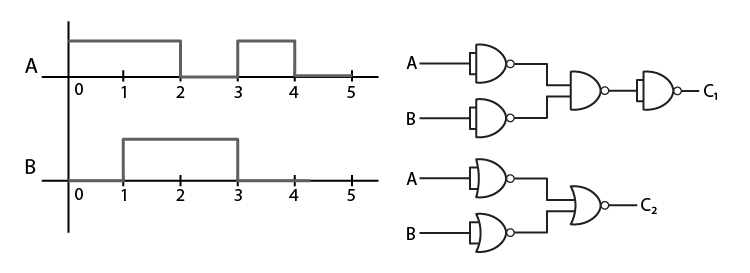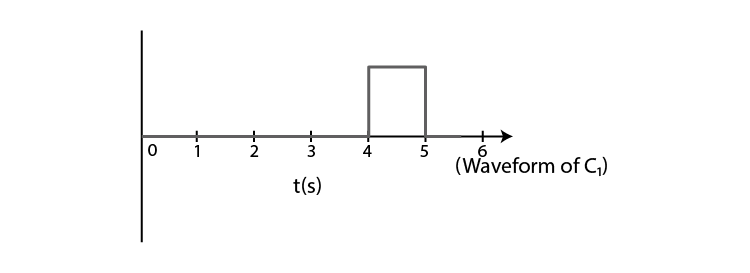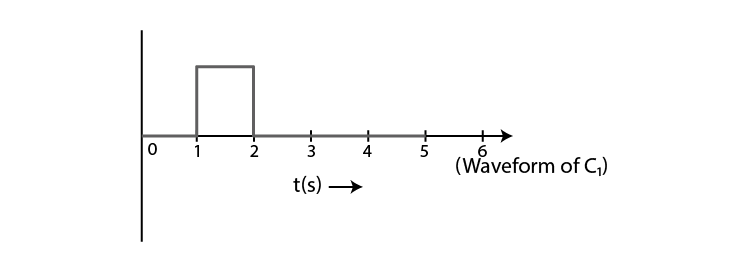14.34 Consider the circuit arrangement in which the input and output characteristics of NPN transistor in CE configuration

Select the values of RB and RC for a transistor whose VBE = 0.7 V, so that the transistor is operating at point Q as shown in the characteristics shown in the figure (b). Given that the input impedance of the transistor is very small and VCC = VBB = 16 V, also find the voltage gain and power gain of circuit making appropriate assumptions.

Vbe = 0.7V

Vcc = Vbb = 16V

Vce = 8V

Ic = 4 mA = 4 × 10-3 A

Ib = 30 × 10-6 A

Rc = 2 kilo ohms

Rb = 510 kilo ohms

Current gain = 1333

Voltage gain = 0.52

Power gain = 69

14.35 Assuming the ideal diode. Explain the waveform.

The waveform of the above circuit would be a sine wave with a dip off in the input wave.

14.36 Suppose a ‘n’-type wafer is created by doping Si crystal having 5 × 1028 atoms/m3 with 1ppm concentration of As. On the surface 200 ppm Boron is added to create the ‘P’ region in this wafer. Considering ni = 1.5 × 1016 m–3,

(i) Calculate the densities of the charge carriers in the n & p regions.

(ii) Comment which charge carriers would contribute largely for the reverse saturation current when the diode is reverse biased.

i) Ne = 5 × 1022 m3

Nh = 0.45 × 1010 m3

Nh = Na = 1 × 1025 m3

Ne = 2.25 × 107 m3

ii) The minority carrier holes of n-region would contribute largely for the reverse saturation current when the diode is reverse biased.

14.37 An X-OR gate has the following truth table:

 A B Y 0 0 0 0 1 1 1 0 1 1 1 0

It is represented by the following logic relation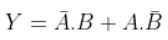Build this gate using AND, OR, and NOT gates.

The logic relation is y = A.B + A.B

14.38 Consider a box with three terminals on top of it:

Three components namely, two germanium diodes and one resistor are connected across these three terminals in some arrangement. A student performs an experiment in which any two of these three terminals are connected in the circuit shown in the figure (b).

The student obtains graphs of current-voltage characteristics for an unknown combination of components between the two terminals connected in the circuit.

The graphs are

(i) when A is positive and B is negative

(ii) when A is negative and B is positive

(iii) When B is negative and C is positive

(iv) When B is positive and C is negative

(v) When A is positive and C is negative

(vi) When A is negative and C is positive

From these graphs of current-voltage characteristic shown in the figures, determine the arrangement of components between A, B and C.

a) A is connected to the n-side of the PN junction and B is connected to the top-side of pn junction.

b) 0.7 V is the knee voltage.

c) Pn junction is connected to C and n-side of pn junction to B and knee voltage is 0.7 V.

d) The above conditions explains the connection of pn-junction along I and II with the resistance.

14.39 For the transistor circuit shown in Fig.14.19, evaluate VE, RB, RE given IC = 1 mA, VCE = 3V, VBE = 0.5 V and VCC = 12 V, β = 100.

Ic = Ie

Rc = 7.8 kilo ohm

Ic (Rc + Re) + Vce = 12

Ve = 1.2 V

Vb = Ve + Vbe = 1.7 V

I = 0.085 mA

Rb = 108 kilo ohms

14.40 In the circuit, find the value of RC.

Ie = Ic + Ib

IcRc + Vce + IeRe = Vcc

Rib + Vbe + IeRe = Vcc

Ib = 11.5/200 mA

Rc + Re = 1.56 kilo ohm

Rc = 560 Ohm

Semiconductor electronics refers to the different electronic circuits, components and devices that are made with a different kind of material, unlike metals and non-metals. Materials based on resistivity and conductivity are categorised into metals, non-metals and semiconductors. They have a wide range of applications, especially in the field of conducting and resisting electricity. Other topics discussed in this chapter are

1. Digital electronics
2. Logic gates
3. Intrinsic and extrinsic semiconductor
4. P-n junction
5. Junction diode as a rectifier

### Why Opt for BYJU’S

BYJU’S is revolutionising the education sector of the country through its interactive and effective learning methodology that uses videos, animations and infographics to teach students. Our interactive model of teaching helps them learn more effectively.